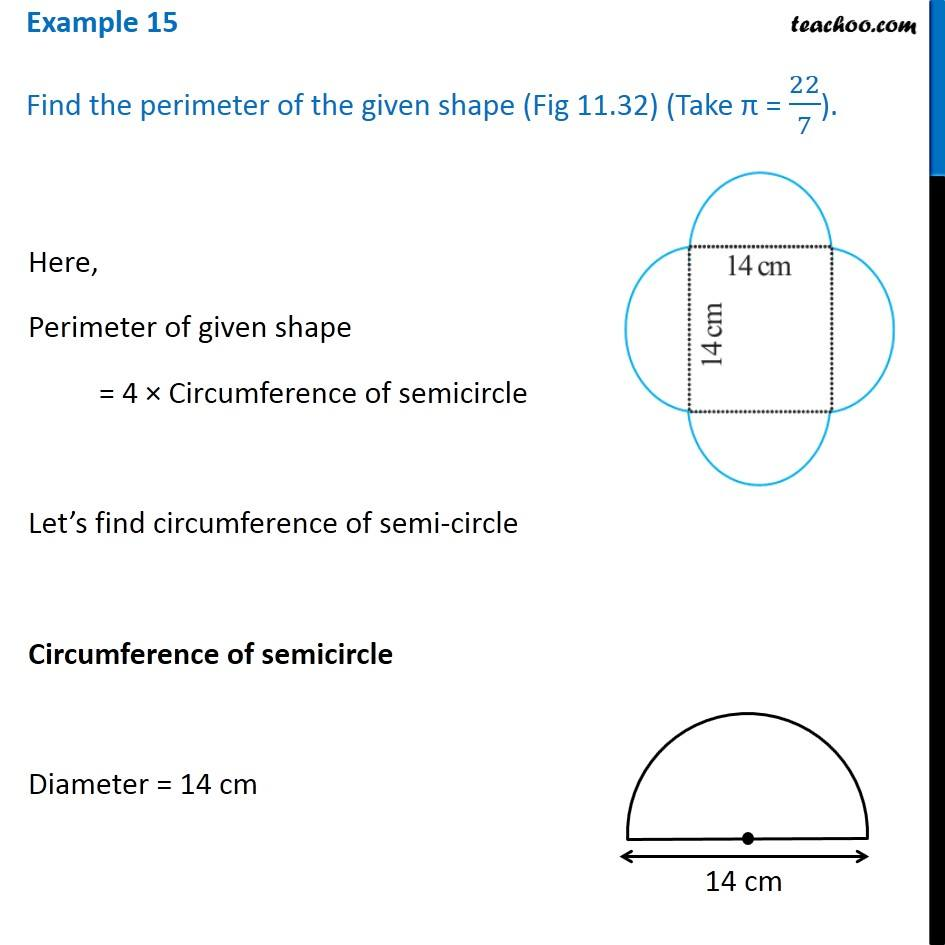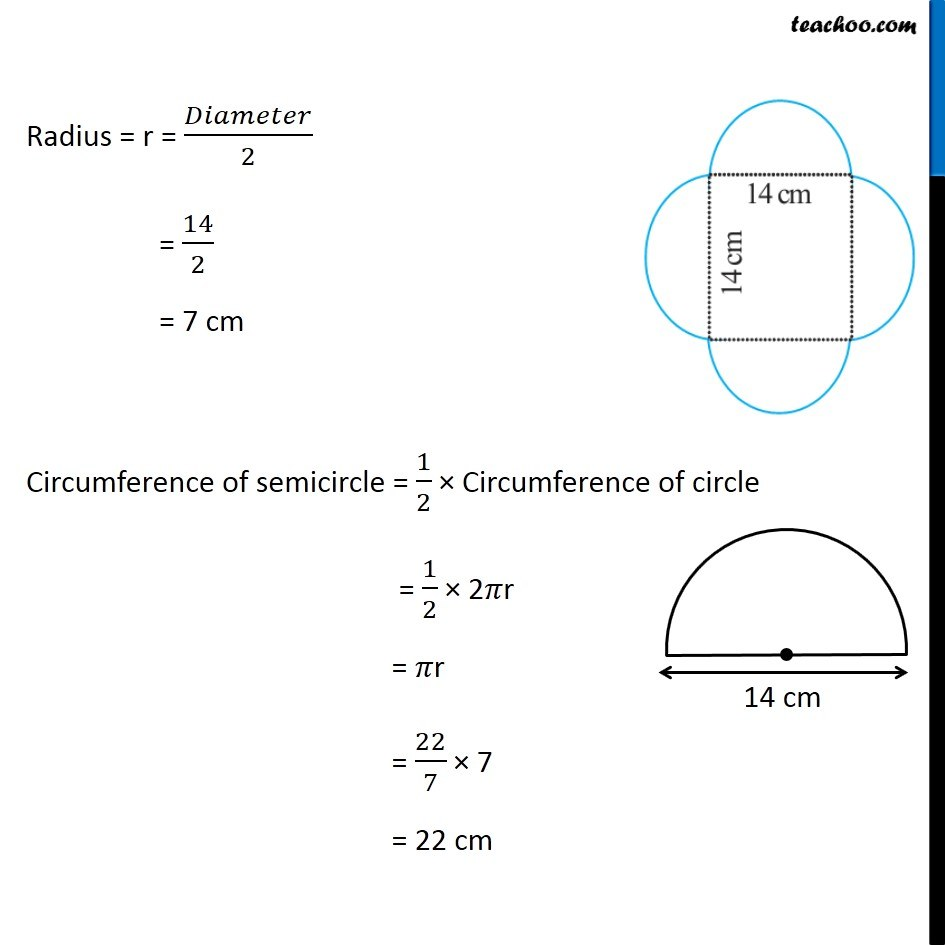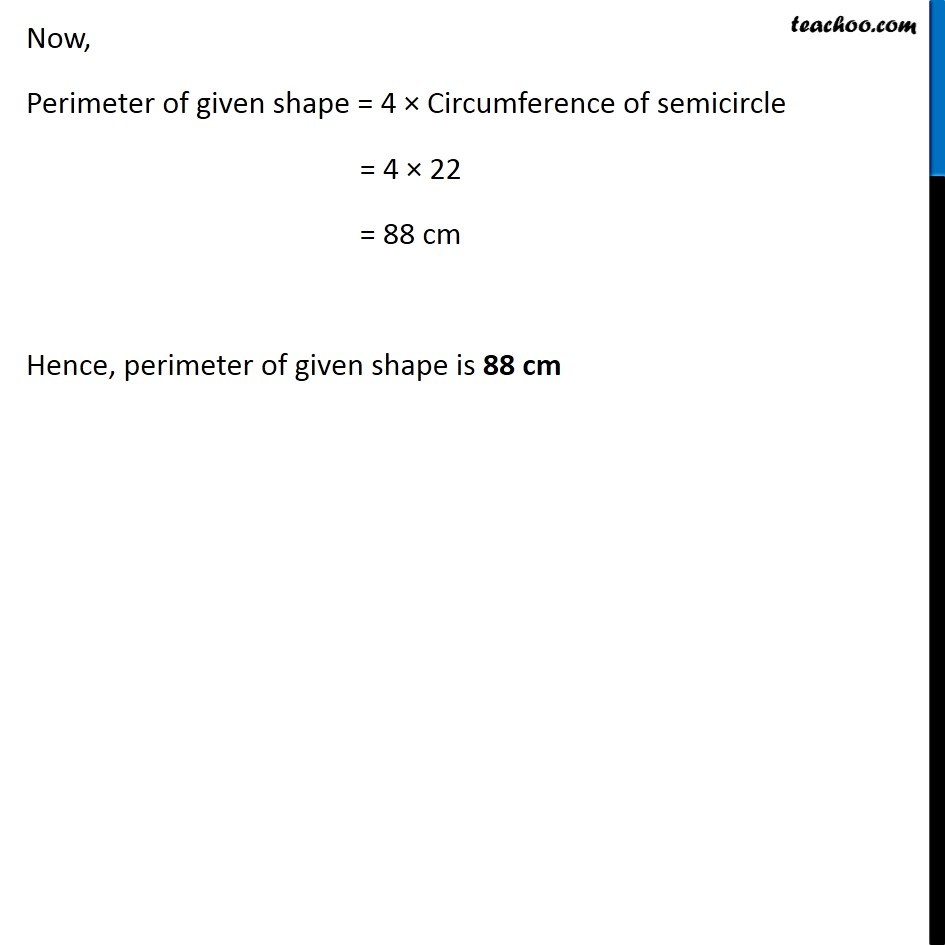1. Chapter 11 Class 7 Perimeter and Area
2. Concept wise
3. Perimeter of a combination of shapes

Transcript

Example 15 Find the perimeter of the given shape (Fig 11.32) (Take π = 22/7). Here, Perimeter of given shape = 4 × Circumference of semicircle Let’s find circumference of semi-circle Circumference of semicircle Diameter = 14 cm Radius = r = 𝐷𝑖𝑎𝑚𝑒𝑡𝑒𝑟/2 = 14/2 = 7 cm Circumference of semicircle = 1/2 × Circumference of circle = 1/2 × 2𝜋r = 𝜋r = 22/7 × 7 = 22 cm Now, Perimeter of given shape = 4 × Circumference of semicircle = 4 × 22 = 88 cm Hence, perimeter of given shape is 88 cm

Perimeter of a combination of shapes